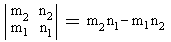# Pick's Theorem Appliesto Farey SeriesRepresent each fraction m/n as a point (n,m) in the Cartesian plane. Draw the grid of lines x = integer and y = integer. A fraction m/n is called visible iff the segment of the straight line connecting the origin with the point (n, m) contains no other grid points.

### Lemma

For n≠0 and m≠0, a point (n,m) is visible iff gcd(n,m) = 1. On the axes, only the points (±1,0) and (0,±1) are visible.### This applet requires Sun's Java VM 2 which your browser may perceive as a popup. Which it is not. If you want to see the applet work, visit Sun's website at https://www.java.com/en/download/index.jsp, download and install Java VM and enjoy the applet.

Imagine now a ray along the positive x-axis. Rotate it counterclockwise and mark all the visible points inside the first octant whose x-coordinate does not exceed, say N. For example, for N = 5, we successively get

0/1, 1/5, 1/4, 1/3, 2/5, 1/2, 3/5, 2/3, 3/4, 4/5, 1/1

which we recognize as the Farey series F5. All other Farey series are obtained in the same manner. First of all, since we are confined to the first octant, all the marked fractions m/n (except of course 1/1) are proper, i.e. m < n. By Lemma, they are also irreducible. Lastly, each fraction equals the slope of the line connecting the corresponding point with the origin. Therefore, they are traversed in the ascending order.

Select any two consecutive points (n1, m1) and (n2, m2) and consider the triangle formed by these points and the origin. Since the two points are visible, the segments connecting them with the origin contain no other lattice points. The segment joining the two can't contain lattice points either because the points were successively traversed by the rotating ray. For the same reason, no lattice point is found inside the triangle. Therefore, by Pick's theorem, the area of the triangle at hand is 1/2.

On the other hand, from Analytic Geometry the area of the triangle is half the absolute value of the determinantThe determinant is positive provided m2/n2 > m1/n1 which is true in our case. Finally, we get ½(m2n1 - m1n2) = ½ which proves the basic property (1) of the Farey series.